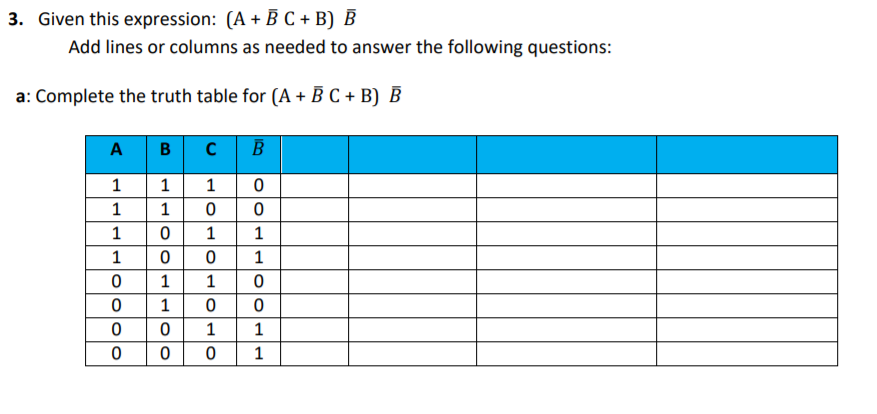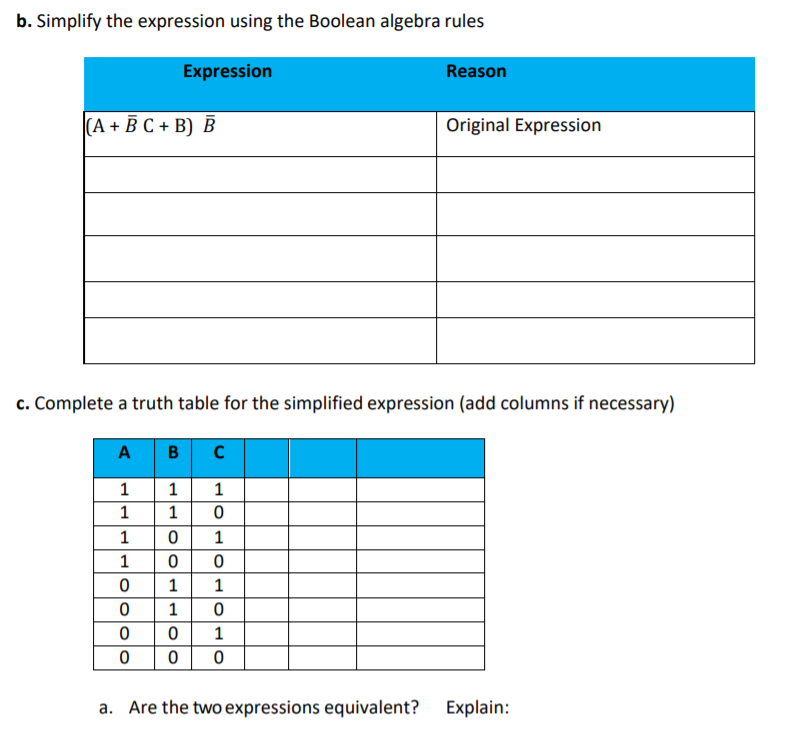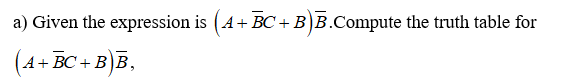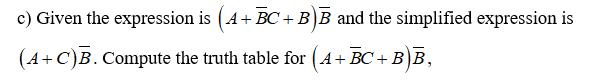# 3. Given this expression: (A + B C + B) BAdd lines or columns as needed to answer the following questions:a: Complete the truth table for (A + B C + B) Bв сA11 b. Simplify the expression using the Boolean algebra rulesExpressionReason(A + Ē C + B) BOriginal Expressionc. Complete a truth table for the simplified expression (add columns if necessary)A B CAre the two expressions equivalent?Explain:a.

Question
1 viewshelp_outlineImage Transcriptionclose3. Given this expression: (A + B C + B) B Add lines or columns as needed to answer the following questions: a: Complete the truth table for (A + B C + B) B в с A 1 1 fullscreenhelp_outlineImage Transcriptioncloseb. Simplify the expression using the Boolean algebra rules Expression Reason (A + Ē C + B) B Original Expression c. Complete a truth table for the simplified expression (add columns if necessary) A B C Are the two expressions equivalent? Explain: a. fullscreen
check_circle

Step 1Step 2

b) Simplify the expression using Boolean algebra rules.

Step 3...

### Want to see the full answer?

See Solution

#### Want to see this answer and more?

Solutions are written by subject experts who are available 24/7. Questions are typically answered within 1 hour.*

See Solution
*Response times may vary by subject and question.
Tagged in

### Math